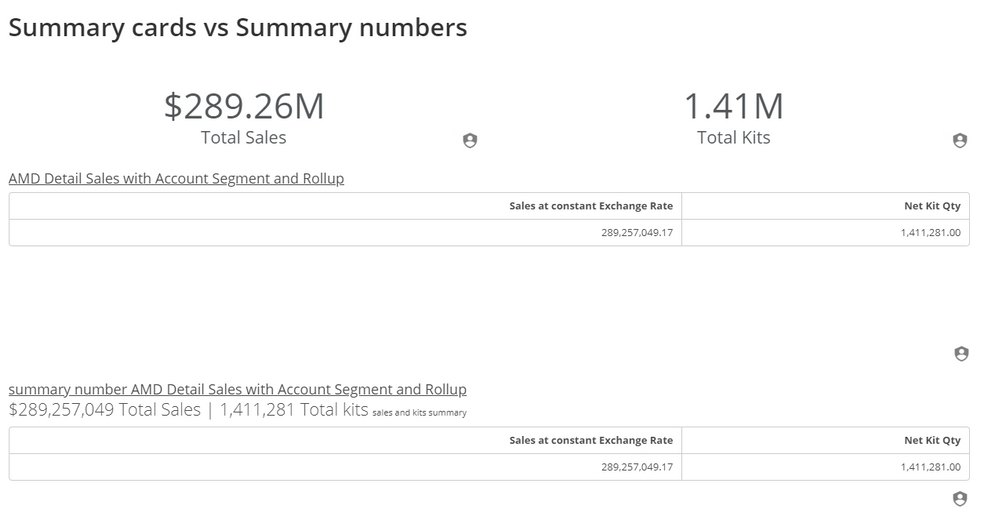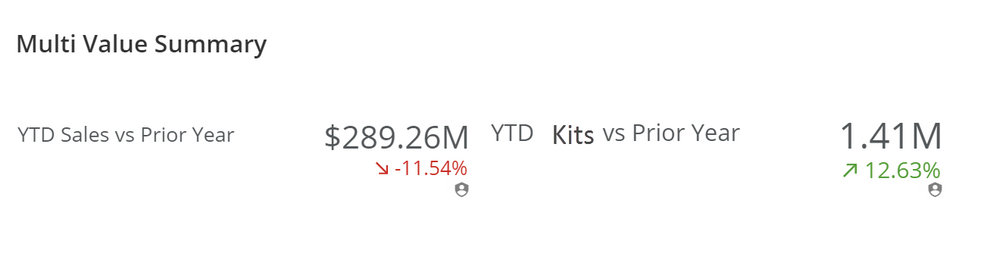# How to display two total numbers, such as spend and savings, on a bar chart.

I have a grouped bar chart that is essentially showing spend and savings broken out by categories such as hotel, food and beverage etc. The summary number displays the total spend. However, my issue is that I need to display the total savings as well. Does anyone know how to do this? It can be in the summary number or the legend area. Thank you for any help.

•CoachYou can display multiple summary numbers by using the concat function along with an aggregate function. It would look something like this:

CONCAT('\$',ROUND(SUM(`YourColumn`),0), ' Total for this column | \$',ROUND(SUM(`YourOtherColumn`),0),' Total for the other column')

The downside is that it won't automatically format your numbers iwth commas, etc. I can post another example of how to account for that if you need to see that.

**Check out my Domo Tips & Tricks Videos

**Make sure toany users posts that helped you.

•CoachYou can display multiple summary numbers by using the concat function along with an aggregate function. It would look something like this:

CONCAT('\$',ROUND(SUM(`YourColumn`),0), ' Total for this column | \$',ROUND(SUM(`YourOtherColumn`),0),' Total for the other column')

The downside is that it won't automatically format your numbers iwth commas, etc. I can post another example of how to account for that if you need to see that.

**Check out my Domo Tips & Tricks Videos

**Make sure toany users posts that helped you.
• Yes! That seems to work. How would you corrrectly format your numbers so that it has commas? Thank you for the answer!

• Yes, please post the example of how to show commas. This was really helpful and worked!

•CoachHere is how you can format numbers into currency values and using the B, M and K abbreviations for billions, millions and thousands since showing the full numbers would make the summary number extremely long.

In this example, the resulting summary number would look like this:

\$10.74M Total Written Premium | \$19.47M Total NLC-MIC Incurred

Here is how you would write your beast mode:

CONCAT('\$'
,CASE
WHEN INSTR(SUM(`Written Premium`),'.') = 13 THEN /*xxx billion*/
WHEN INSTR(SUM(`Written Premium`),'.') = 12 THEN /*xx billion*/
WHEN INSTR(SUM(`Written Premium`),'.') = 11 THEN /*x billion*/
WHEN INSTR(SUM(`Written Premium`),'.') = 10 THEN /*xxx million*/
WHEN INSTR(SUM(`Written Premium`),'.') = 9 THEN /*xx million*/
WHEN INSTR(SUM(`Written Premium`),'.') = 8 THEN /*x million*/
WHEN INSTR(SUM(`Written Premium`),'.') = 7 THEN /*xxx thousand*/
WHEN INSTR(SUM(`Written Premium`),'.') = 6 THEN /*xx thousand*/
WHEN INSTR(SUM(`Written Premium`),'.') = 5 THEN /*x thousand*/
END
,' Total Written Premium | \$'

,CASE
WHEN INSTR(SUM(`TotalIncurredForYear`),'.') = 13 THEN /*xxx billion*/
CONCAT(LEFT(SUM(`TotalIncurredForYear`),3),'.',SUBSTRING(SUM(`TotalIncurredForYear`),4,2),'B')
WHEN INSTR(SUM(`TotalIncurredForYear`),'.') = 12 THEN /*xx billion*/
CONCAT(LEFT(SUM(`TotalIncurredForYear`),2),'.',SUBSTRING(SUM(`TotalIncurredForYear`),3,2),'B')
WHEN INSTR(SUM(`TotalIncurredForYear`),'.') = 11 THEN /*x billion*/
CONCAT(LEFT(SUM(`TotalIncurredForYear`),1),'.',SUBSTRING(SUM(`TotalIncurredForYear`),2,2),'B')
WHEN INSTR(SUM(`TotalIncurredForYear`),'.') = 10 THEN /*xxx million*/
CONCAT(LEFT(SUM(`TotalIncurredForYear`),3),'.',SUBSTRING(SUM(`TotalIncurredForYear`),4,2),'M')
WHEN INSTR(SUM(`TotalIncurredForYear`),'.') = 9 THEN /*xx million*/
CONCAT(LEFT(SUM(`TotalIncurredForYear`),2),'.',SUBSTRING(SUM(`TotalIncurredForYear`),3,2),'M')
WHEN INSTR(SUM(`TotalIncurredForYear`),'.') = 8 THEN /*x million*/
CONCAT(LEFT(SUM(`TotalIncurredForYear`),1),'.',SUBSTRING(SUM(`TotalIncurredForYear`),2,2),'M')
WHEN INSTR(SUM(`TotalIncurredForYear`),'.') = 7 THEN /*xxx thousand*/
CONCAT(LEFT(SUM(`TotalIncurredForYear`),3),'.',SUBSTRING(SUM(`TotalIncurredForYear`),4,2),'K')
WHEN INSTR(SUM(`TotalIncurredForYear`),'.') = 6 THEN /*xx thousand*/
CONCAT(LEFT(SUM(`TotalIncurredForYear`),2),'.',SUBSTRING(SUM(`TotalIncurredForYear`),3,2),'K')
WHEN INSTR(SUM(`TotalIncurredForYear`),'.') = 5 THEN /*x thousand*/
CONCAT(LEFT(SUM(`TotalIncurredForYear`),1),'.',SUBSTRING(SUM(`TotalIncurredForYear`),2,2),'K')
END

,' Total NLC-MIC Incurred')

**Check out my Domo Tips & Tricks Videos

**Make sure toany users posts that helped you.
• Great! Thank you so much!

•Coachyou could also use gauge cards and the story dashboard to provide multiple summaries...“There is a superhero in all of us, we just need the courage to put on the cape.” -Superman
This discussion has been closed.